The Golden Rectangle

A rectangle can be divided in two pieces: a square with the smaller side and another rectangle.

For a certain proportion of the sides of the initial rectangle, by that procedure we obtain a similar rectangle to the previous one.

Then we have a golden rectangle.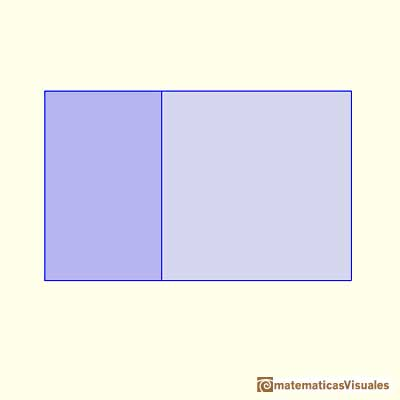From Euclid's definition of the division of a segment into its extreme and mean ratio we introduce a property of golden rectangles and we deduce the equation and the value of the golden ratio.

If we start from a golden rectangle that procedure can be repeated indefinitely.This golden or divine proportion can be expressed in this way: the ratio of the larger part to the smaller is equal to the ratio of the whole to the larger part.

Then we obtain the golden numberThis infinite process suggests that the sides of a golden rectangle are incommensurable or, otherwise, that the golden number is irrational.

We can see that rectangle ABDF and rectangle CDFH are similar and that CDFH is rotated (a quarter-turn)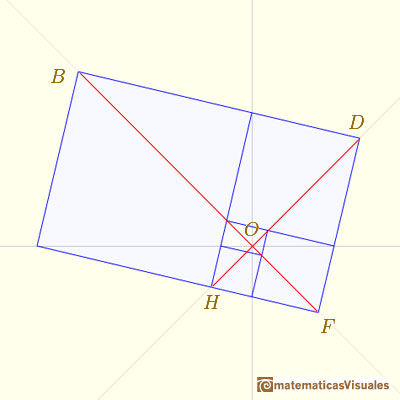Then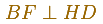We call O the intersection point.

Now we can consider the line OC: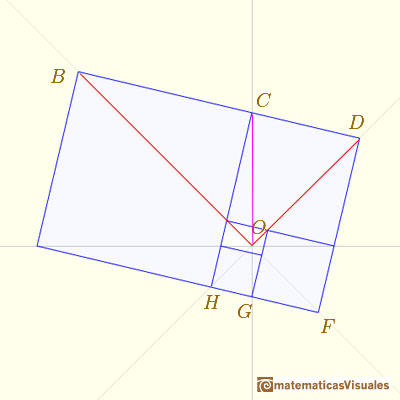AsThen OC bisects the (right) angle BODSimilarlyO is in the line CG. The same for AE and thenThese four straight lines, orthogonal in pairs, they contain all the vertices of those infinite rectangles. Each one of these pairs of straight lines bisects the other pair.REFERENCES

Coxeter H. S. M. - Introduction to Geometry (John Whiley and Sons, Second Edition, 1969)The diagonal of a regular pentagon are in golden ratio to its sides and the point of intersection of two diagonals of a regular pentagon are said to divide each other in the golden ratio or 'in extreme and mean ratio'.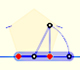You can draw a regular pentagon given one of its sides constructing the golden ratio with ruler and compass.In his book 'Underweysung der Messung' Durer draw a non-regular pentagon with ruler and a fixed compass. It is a simple construction and a very good approximation of a regular pentagon.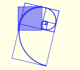The golden spiral is a good approximation of an equiangular spiral.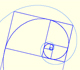Two equiangular spirals contains all vertices of golden rectangles.The twelve vertices of an icosahedron lie in three golden rectangles. Then we can calculate the volume of an icosahedronSome properties of this platonic solid and how it is related to the golden ratio. Constructing dodecahedra using different techniques.The first drawing of a plane net of a regular dodecahedron was published by Dürer in his book 'Underweysung der Messung' ('Four Books of Measurement'), published in 1525 .He studied transformations of images, for example, faces.One eighth of a regular dodecahedon of edge 2 has the same volume as a dodecahedron of edge 1.Leonardo da Vinci made several drawings of polyhedra for Luca Pacioli's book 'De divina proportione'. Here we can see an adaptation of the dodecahedron.In an equiangular spiral the angle between the position vector and the tangent is constant.Two transformations of an equiangular spiral with the same general efect.A Dilative Rotation is a combination of a rotation an a dilatation from the same point.There is a standarization of the size of the paper that is called DIN A. Successive paper sizes in the series A1, A2, A3, A4, and so forth, are defined by halving the preceding paper size along the larger dimension.